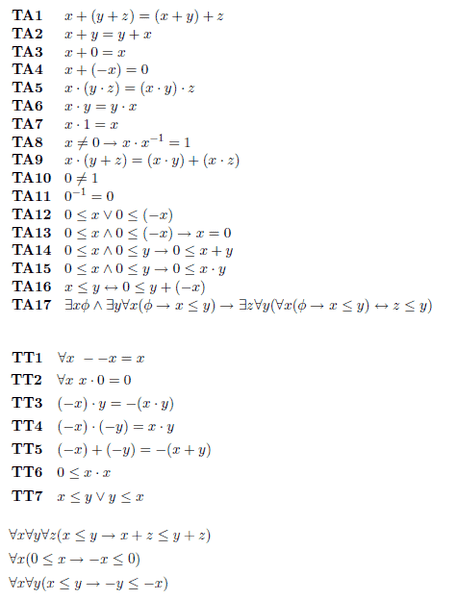# [LOGIC] Prove if y + (-x) = 0 then y = x

y + (-x) = 0 → y = x

Need to do this in Tarski arithmetic, axioms below, as well as results below that which have been previously provedI really have no idea how I can move this x over, can anyone help me out with a starting axiom?

Is it just TA4 that implies y=x? That simple? If it is then is it possible to do it another way with a bit more rigour?

Thanks

Last edited:

HallsofIvy
Homework Helper
Saying that y+ (-x)= 0 is the same as saying that -x is the additive inverse of y as well as of x (which is how "-x" is defined- That's TA4). But you cannot use TA4 only because TA4 alone does not say there might not be that two different numbers have the same additive inverse. What you need to do is show two other things:
1) that if -x is the additive inverse of x then x is the additive inverse of -x.
2) every number has a unique additive inverse.
You should be able to show those from the given axioms.

However, I am really concerned about "TA11" which appears to say that the multiplicative inverse of 0 is 0. That would imply that 0*0= 1 which is not true. It is, of course, true that -0= 0. Perhaps that was what was intended.

Saying that y+ (-x)= 0 is the same as saying that -x is the additive inverse of y as well as of x (which is how "-x" is defined- That's TA4). But you cannot use TA4 only because TA4 alone does not say there might not be that two different numbers have the same additive inverse. What you need to do is show two other things:
1) that if -x is the additive inverse of x then x is the additive inverse of -x.
2) every number has a unique additive inverse.
You should be able to show those from the given axioms.

However, I am really concerned about "TA11" which appears to say that the multiplicative inverse of 0 is 0. That would imply that 0*0= 1 which is not true. It is, of course, true that -0= 0. Perhaps that was what was intended.

TA11 my lecturer mentioned

'Note that all function symbols have to be defined as total, and therefore 0−1 has to be assigned a meaning even though in reality the number 0 has no reciprocal; axioms TA8 and TA11 in effect define x−1 as the reciprocal of x when x ≠ 0, and 0 otherwise. This does not interfere with the correct functioning of the system. Axiom TA11 is really only there to ensure that the axiom set as a whole defines a categorical theory, that is, that it has a unique model up to isomorphism.'

for 1) (some trivial steps omitted)

x + (-x) = 0
x + (-x) + x + (-x) = 0
commute some stuff around and get
(-x) + x = 0

for 2)

How do I start?

Thanks

It's been many years since I have done proofs like this. Seems like you are going to be using TA2 and TA4. I am just not sure what you are allowed to substitute and use the axioms.
like if I look at y+(-x) could I use TA2 and get y+(-x) = (-x) + y
or can I set 0 = x+(-x) using TA4
giving me (-x)+y = x+(-x) does that imply that x=y using TA2?
Or do you need to do it someway so that at the end of just substituting in the stuff below that it gives you y=x?

It's been many years since I have done proofs like this. Seems like you are going to be using TA2 and TA4. I am just not sure what you are allowed to substitute and use the axioms.
like if I look at y+(-x) could I use TA2 and get y+(-x) = (-x) + y
or can I set 0 = x+(-x) using TA4
giving me (-x)+y = x+(-x) does that imply that x=y using TA2?
Or do you need to do it someway so that at the end of just substituting in the stuff below that it gives you y=x?

On the last step I need to be able to justify why we are removing the (-x) using the axioms only

If I had a lemma that said

y+z=x+z → y=x

then that way would be fine

But I don't, so essentially now I'm trying to prove this lemma

That was what I was wondering, so you can't just say since a+b = b+a and a = -x, then b = y = x. Been to long to know what you can and can't do, so I guess I won't be helping much haha.